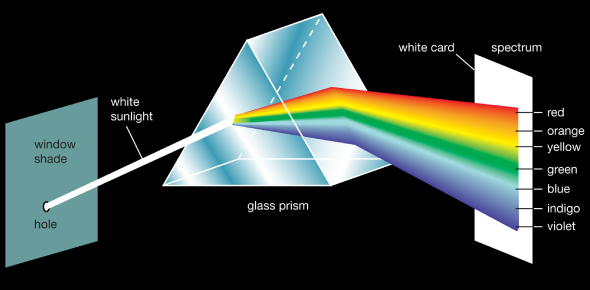# Light Test: Physics Quiz! Trivia

19 Questions | Attempts: 2202
ShareSettings.

• 1.
Only the wave theory of light offers an explanation for the ability of light to exhibit.
• A.

Interference

• B.

Reflection

• C.

Photoelectric emission

• D.

• 2.
Which two characteristics of light can best be explained by the wave theory of light?
• A.

Relection and refraction

• B.

Reflection and interference

• C.

Refraction and diffraction

• D.

Interference and diffraction

• 3.
Which phenomenon can be observed for transverse waves only?
• A.

Reflection

• B.

Diffraction

• C.

Polarization

• D.

Refraction

• 4.
Sources that produce waves with a constant phase relation are said to be:
• A.

Polarized

• B.

Diffused

• C.

Refracted

• D.

Coherent

• 5.
If all parts of a light beam have a constant phase relationship, with the same wavelength and frequency, the light beam is
• A.

Monochromatic and coherent

• B.

Mono and incoherent

• C.

Polychromatic and coherent

• D.

Poly and incoherent

• 6.
The diffraction pattern produced by a double slit will show greatest separation of maxima when the color of the light source is
• A.

Red

• B.

Orange

• C.

Blue

• D.

Green

• 7.
If the wavelength of the light is decreased, the width of the central maximum in the diffraction pattern will
• A.

Decrease

• B.

Increase

• C.

Remain the same

• 8.
A wave is diffracted as it passes through an opening in a barrier. The amount of diffraction that the wave undergoes depends on both the
• A.

Amplitude and frequency of the incident wave

• B.

Wavelength and speed of the incident wave

• C.

Wavelength of the incident wave and size of the opening

• D.

Amplitude of the incicdent wave and the size of the opening

• 9.
If the screen is moved closer to the slits, the distance between the central maximum and the first maximum will
• A.

Decrease

• B.

Increase

• C.

Remain the same

• 10.
If the distance between the slits is decreased, the distance between the central max and the first max will
• A.

Decrease

• B.

Increase

• C.

Remain the same

• 11.
The photon model of light is more appropriate than the wave model in explaining
• A.

Interference

• B.

Refraction

• C.

Polarization

• D.

Photoelectric emission

• 12.
Light demonstartes the characteristics of
• A.

Particles only

• B.

Waves only

• C.

Both particles and waves

• D.

Neither

• 13.
Interference and diffraction can be explained by:
• A.

The wave theory only

• B.

The particle theory only

• C.

Neither

• D.

Both

• 14.
The color of visible light is determined by its:
• A.

Frequency

• B.

Amplitude

• C.

Intensity

• D.

Speed

• 15.
Increasing the amplitude of electromagnetic radiation increases its
• A.

Frequency

• B.

Speed

• C.

Intensity

• D.

Period

• 16.
Compared to the period of red light, the period of violet light is
• A.

Less

• B.

Greater

• C.

Same

• 17.
Compared to the period of a wave of red light, the period of a wave of blue light is
• A.

Less

• B.

Greater

• C.

Same

• 18.
Electrons oscillating with a frequency of 2.0X10 10 hertz produce electromagnetic waves. These waves would be classified as
• A.

Infrared

• B.

Visible

• C.

Microwave

• D.

X-ray

• 19.
Which pair of terms best describes light waves traveling from the Sun to Earth?
• A.

Electromagnetic and transverse

• B.

Electromagnetic and longitudinal

• C.

Mechanical and transverse

• D.

Mechanical and longitudinal

## Related TopicsBack to top
×

Wait!
Here's an interesting quiz for you.